Answers to the review problems for the second exam, 251:05-10 in spring 2006

Problems with letters whose backgrounds are this color have
answers here. Those with backgrounds in this color do not.

Have you done a problem?

D
 A B C E F G H I J K L M N O P QIn the diary R S T U Va) only W X

Answers contributed by section
5678910
64122.51

A
Contributed by Robert J. Comito of Section 5:

Sketch the region described in spherical coordinates by 0<=phi<=Pi/3 and 0<=rho cos(phi)<=2 and 0<=<=2Pi.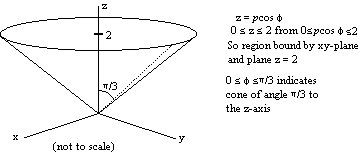B
Contributed by Chirag Walawalkar of Section 9:

Let f(x,y)=1/x+1/y+xy. True or false? Explain BRIEFLY:
a) f has a local maximum at (1,1).
b) f has a saddle point at (1,-1).

a. (1, 1) is Local max?
First we take the first derivative with respect to x and then with respect to y:
fx(x,y)=-1/x^2+y fx(1,1)=0
fy(x,y)=-1/y^2+x fy(1,1)=0
Therefore (1,1) is a critical point.
Now we do the second derivative test (S.D.T)
fxx(x,y)=2/x^3, fyy(x,y)=2/y^3 fxy(x,y)=1
H=fxx(1,1)fyy(1,1)-[fxy(1,1)^2]
H=(2)(2)-1
H=3
By S.D.T since H>0 and fxx(1,1)>0 f has a local min at (1,1) therefore the answer is False.

b) fx=-1/x2+y ==> fx(1,-1)=-1+-1=-2 not zero.
it is not a critical point therefore statement b) is also false.

C
Contributed by Shubha Sarode of Section 6:

Find the absolute max and min of f(x,y)=x2+2x+2y2 on the circle G(x,y)=x2+y2=4.

We use Lagrange multipliers to solve the equation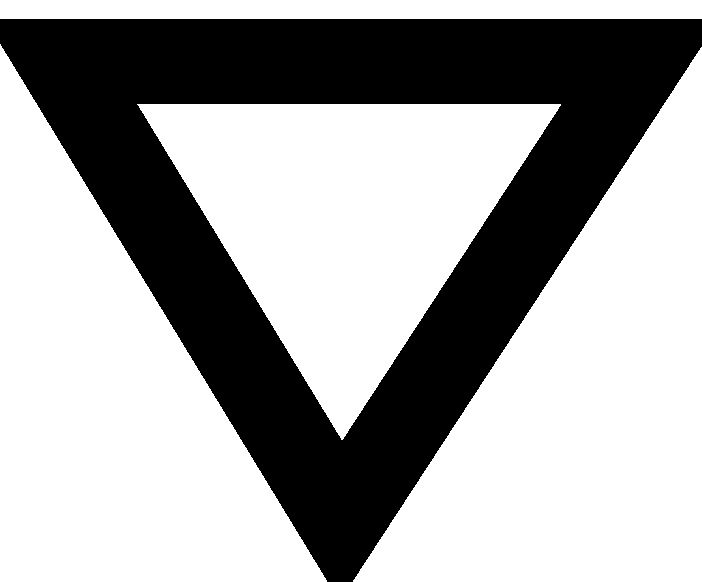F=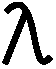G:
Fx=2x+2, Gx=2x, Fy=4y, and Gy=2y
2x+2=2x
4y=2y Note:can equal 2 or y=0
Ifequals 2, then we plug this value in the equation 2x+2=2x and we get 2x+2=4x. Solving this equation we get x=1. If we plug this value into G(x,y)=4 we get y=+/-sqrt(3).
Therefore possible max/min's could occur at (1,sqrt(3)) and (1,-sqrt(3)). We need to evaluate these points in F(x,y) to see their values. Plugging in both sets of points, we get the value of 9.
Now we need to see what the possible points could be if y=0. Plugging in y=0 in the equation x2+y2=4 we get the x values of +2 and -2. Therefore, possible points are (2,0) and (-2,0) Evaluating F(x,y) at these points we get 8 and 0 respectively.
so:
(1,sqrt(3)) and (1,-sqrt(3)) gives F=9, This is the max.
(2,0) gives 8.
(-2,0) gives 0. This is the min.

b) To find the max and min on the disk x2+y2 less then or equal to 4 we just need to compare the values of F at the critical points inside the disk with values at the points on the boundary.
The critical points are:
Fx=2x+2 therefore x=-1; Fy=2y therefore y=0.
The value of F(x,y) at (-1,0) is -1.

Therefore, -1 is going to be the absolute min and 9 (from above) is going to stay as the absolute max.

Comment Please note that in this solution, tracing down every alternative (including what happens if some variable is 0) was necessary to get the answer. You can't just assume all or any of the relevant variables and not 0.

D
Contributed by Jennifer Ng of Section 22 in fall 2008: please see here.
An error was found by S. Speights (4/11/2010). The Jacobian for spherical coordinates should be ρ2sin(φ), so the solution should have another power of ρ and the final answer should therefore be (4/15)Π.

E
Contributed by Sonam Patel of Section 5:

Find all the critical points of F(x,y)=x^2-4xy+y^3-3y and describe the type of each critical point.

Fx=2x-4y and Fy=-4x+3y^2-3

Now, set both equations equal to zero in order to find the critical points:
x=2y and -4x+3y^2-3=0 by substitution you get, -4(2y)+3y^2-3=0 which can be also written as 3y^2-8y-3=0. When factored, you get (3y+1)(y-3)=0 and therefore y=-1/3 or y=3.
When y=3, x=6. So the first critical point is (6,3). When y=-1/3,x=-2/3, and so the second critical point is (-2/3,-1/3).

In order to classify them, take second partial derivatives. Fxx=2 , Fxy=-4, Fyy=6y, Fyx=-4 D=D(a,b)=Fxx(a,b)Fyy(a,b)-[Fxy(a,b)]2=12y-16 so D(6,3)=20 and Fx(6,3)=2. When D(a,b)>0 and Fx(a,b)>0, then F(a,b) is a local minimum. Therefore (6,3) is a local minimum of F.
D(-2/3,-1/3)=-20. D(-2/3,-1/3)<0 and therefore D(-2/3,-1/3) is a saddle point.

Comment The solution by Sonam Patel arrived at Sat, 1 Apr 2006 15:24:14. A similar solution with the same answers by Demetri Koloseus-Gagnon arrived at Sat, 1 Apr 2006 15:34:33. The competition is heating up! Thanks to both of these people.

F
Contributed by Adam Skrzypczak of Section 10:

Find the maximum and minimum values of x-4y+2z subject to the constraint that (x,y,z) lies on the ellipsoid x2+y2+5z2=1.

F(x,y,z) =x-4y+2z and G(x,y,z)=x2+y2+5z2=1F=G (for diff. funct x, y, z) so you get 4 equations:
1. 1=(2x)
2. -4=(2y)
3. 2=(10z)
4. x2+y2+5z2=1cannot be 0 because the first equation would then be 1=0.
Solve for each of the variables and insert into the constraint equation to get:
1/(42)+4/(2)+1/(52)=1 so=sqrt(89/20).
Then plug back into eq's 1 through 3 and find values for x,y,z :
x=(+/-)1/[2sqrt(89/20)] and y=(+/-)-2/sqrt(89/20) and z=(+/-)1/[5sqrt(89/20)]
which gives you two points:
(1/2sqrt(89/20),-2/sqrt(89/20),1/5sqrt(89/20)) And (-1/2sqrt(89/20),2/sqrt(89/20),-1/5sqrt(89/20)) Then plug back into F(x,y,z) and you find your max and min! Max occurs at (1/2sqrt(89/20),-2/sqrt(89/20),1/5sqrt(89/20)) and is sqrt(89/20)([1/2]+8+[2/5]).
Min occurs at (-1/2sqrt(89/20),2/sqrt(89/20),-1/5sqrt(89/20)) and is -sqrt(89/20)([1/2]+8+[2/5]).

G
Contributed by Demetri Koloseus-Gagnon of Section 6:

Change the order of integration in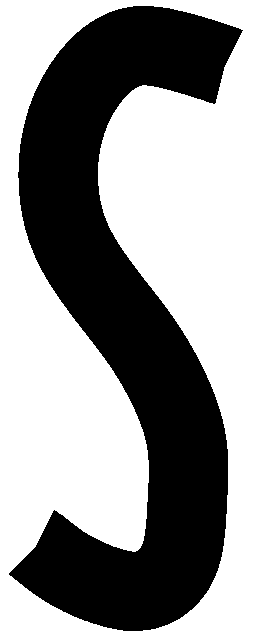120ln yf(x,y) dx dy.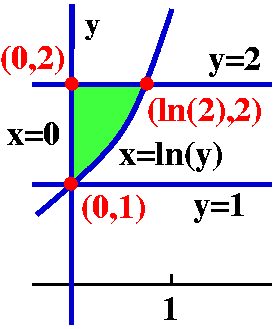0ln(2)ex2f(x,y) dy dx.

Comment Certainly the answer is correct. I remark, though, that an answer alone may not receive full credit. In this case, some explanation is needed. Probably a picture together with some algebra is best. For example, one boundary curve of the problem statement is x=ln(y) which turns into y=ex. The picture to the right of the region of integration should show enough that a reader of the problem solution is convinced the student understands the problem.

H
Contributed by Robert J. Comito of Section 5: Change the integral030sqrt(9-y2)sqrt(x2+y2)sqrt(18-x2-y2)(x2+y2+z2) dz dx dy to spherical coordinates. DON'T EVALUATE THE INTEGRAL.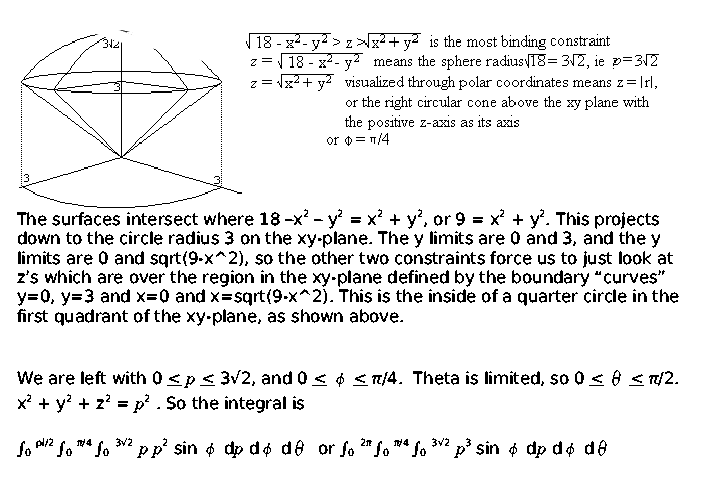Comment This has been corrected today (4/5/2006) as a result of e-mail from Karin Jensen of Section 6. One of the previouslimits was incorrect. This "solid" only is in the first octant, above a quarter circle (quarter disc!) in the first quadrant of the xy-plane. I thank Ms. Jensen for her careful reading!
There;s also an error in the integrand, as Michael Boxer of section 6 notices. Since rho2=xs2+y2+z2, the integrand should be rho4. I am sorry, and thank Mr. Boxer.

I
Contributed by Robert J. Comito of Section 5:

a) Compute231/xx2x2y-2x dy dx.
b) Write this iterated integral in ``dx dy'' order. You may want to begin by sketching the area over which the double integral is evaluated. You are not asked to evaluate the dx dy result, which may be one or more iterated integrals.Comment Very neat answer. Thank you.
Of course we can compute the dx dy version and see if the answer is the same as the answer to a), or we can type and read.
>int(int(x^2*y-2*x,x=1/y..3),y=1/3..1/2) + int(int(x^2*y-2*x,x=2..3),y=1/2..4) + int(int(x^2*y-2*x,x=sqrt(y)..3),y=4..9);

1625
----
14

K
Contributed by Sejalkumari Patel of Section 7:

CalculateDy dA where D is bounded by y=x-1 and y2=2x+6.

The line y=x-1 intersects the curve y2=2x+6 at two places, so we can two points (-1,-2) and (5,4). You can find these two points by plugging in the value of x=y+1 into the formula y2=2x+6. This becomes y2=2(y+1)+6=2y+8, so y2-2y-2=0 and (y+2)(y-4)=0.The limits of x and y are:
(y2-6)/2<=x<=y+1 and -2<=y<=4.
The double integral becomes the iterated integral:-24(y2-6)/2y+1y dx dy=-24 xy](y2-6)/2y+1.
After some algebra, this is-24-2(y2-y3/2+4y)dy= -2(y3/3-y4/8+2y2)]-24=18.

Comment Not out of disbelief, but sheerly because everyone should wear both suspenders and a belt, I present:

> int(int(y,x=(y^2-6)/2..y+1),y=-2..4);
18

L
Contributed by Qi Wen of Section 6:

Let F(x,y)=e2yi+(1+2xe2yj. Find a function f(x,y) such thatf=F and use it to evaluateCdr where r(t)=sqrt(t)i+(1+t3)j, and 0=<t<=1.

fx=e2yfxdx=e2ydx=xe2y+g(y)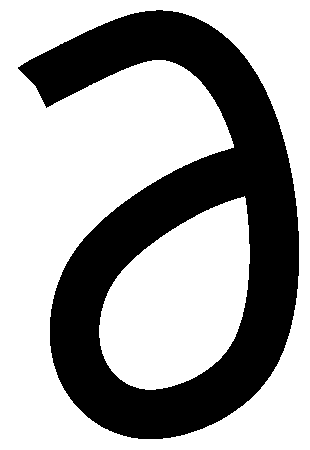[xe2y+g(y)]/y=2x e2y+g´(y).
The original function for the j component is 1+2x e2y,therefore the g'(y) is 1, then g(y)=y.
f(x,y)=xe2y+y

Because r(t)=sqrt(t)i+(1+t3)j and 0<=t<=1. Therefore the start and the end points are (0,1) and (1,2).Cdr=f(x2,y2)-f(x1,y1) and f(x2,y2)-f(x1,y1)=f(1,2)-f(0,1) and f(1,2)-f(0,1)=e4+1.

O
Contributed by Steve Swern of Section 8: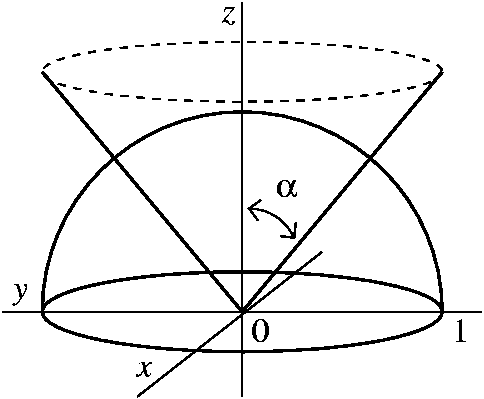In this problem H is the upper half of the unit sphere in R3: those (x,y,z) with x2+y2+z2<=1 and z>=0. There is a right circular cone whose vertex is (0,0,0) and whose axis of symmetry is the positive z-axis which divides the volume of H into two equal parts. Find the angle alpha that determines this cone. The diagram defines alpha, which is the angle that the positive z-axis makes with a line on the cone through the vertex.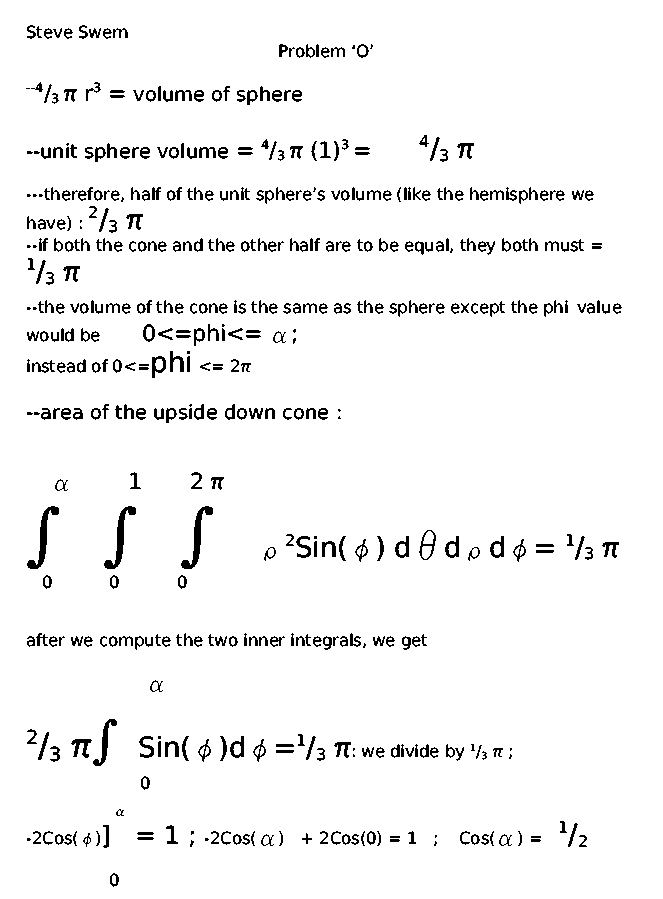P
Contributed by Steve Swern of Section 8:

Suppose D is the path consisting of three straight line segments, first from (1,2) to (4,-3), then from (4,-3) to (2,6), and then from (2,6) to (3,4). ComputeD(2xy3) dx+(3x2y2+4y3) dy.

Since we're computing a work line integral for a vector field that is CLEARLY the gradient of another function. We can look at it and figure out what the potential was...

We'll use the dy side since it's more defining: 3(x2)y2+4y3dy ==> a little antidifferentiation ==> f(x,y)=x2(y3)+y4
this checks out for the dx side as well because fx=2xy3.
given on the formula sheet is this :
"For V conservative with potential f (we were given V and i showed you how to find f).. the integral for V .. blah blah blah... = f(THE END) - f(THE START)"
the start was (1,2).. that danced around a couple points... and ended at (3,4) ...f(3,4)-f(1,2)=832-24=808

Comment Mr. Swern's rather "breezy" approach is correct. You might worry about the curve being defined in several pieces, but things do work out. Certainly each line segment can use the potential, so that the total integral is equal to this sum:
f(3,4)-f(2,6) (from the third line segment) + f(2,6)-f(4,-3) (from the second line segment) + f(4,-3)-f(1,2) (from the first line segment)
and the sum "telescopes" to give f(3,4)-f(1,2), even as asserted by Mr. Swern.

R
Contributed by Robert J. Comito of Section 5: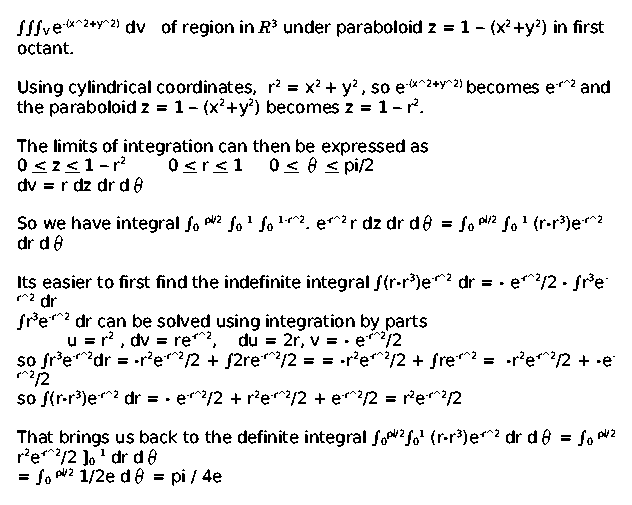S
Contributed by Jocelyn Alexander of Section 5:

Let C be the straight line segment from (0,1) to (4,3). FindCx2y ds.

Vector representation of line segment that starts at r0 and ends at r1:
r(t)=(1-t)r0+t*r1
For the line segment given, r0=(0,1) and r1=(4,3) r(t)=(1-t)<0,1>+t<4,3>=<0+4t,1-t+3t>=<4t,1+2t>.
Parametric representation:
x=4t so dx/dt=4
y=1+2t so dy/dt=2
(0<=t<=1)
ds=sqrt[(dx/dt)2+(dy/dt)2]dt=sqrt(42+22)dt=sqrt(16+4)dt=sqrt(20)dtCx2y ds=t=0t=1(4t)2(1+2t)sqrt(20)dt=sqrt(20)01(16t2+32t3)dt=sqrt(20)[16t3/3+8t4]01=([16/3]+8)2(sqrt(5))

T
Contributed by Chirag Walawalkar of Section 9: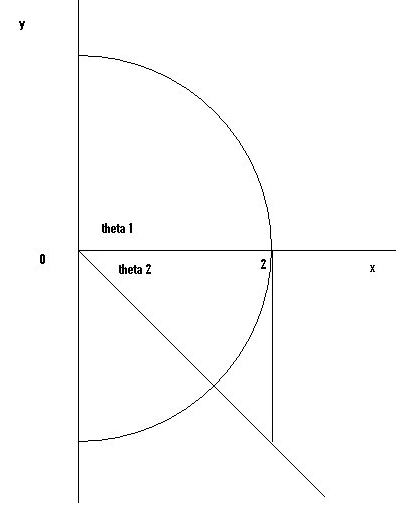ComputeRx dA where R is the region to the right of the y-axis and bounded by the circle of radius 2 centered at the origin, the positive part of the y-axis and the line y=-x.

R bounded by the positive y axis and the circle of radius 2 centered at origin positive x axis and the line y=-x.1 is the angle between the positive x-axis and the positive y-axis. It is Pi/2.2 is the angle between the positve x axis and the line y=-x. We can find this angle using the picture and the fact that the line cuts the quarter circle in half thus theta 2 will be half of Pi/2 or Pi/4.
We can also find2 using the fact that y=-x and when x=2, y must equal -2, and2=arctan(-2/2)=-Pi/4. The negative sign occurs because the angle is in the 4th quadrant (the picture is better here). r is simply between 0 and 2.
x=r cos() and dA=r dr dso the integral becomes-Pi/4Pi/202r cos() r drd=(-Pi/4Pi/2cos()d)(02r2dr)=(r3/3]02)(sin()]-Pi/4Pi/2=(8/3)(1+sqrt(2)/2) (

U
Contributed by Tina Lee of Section 6: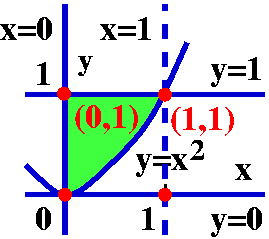change x2<y<1 and 0<x<1 to 0<y<1 and 0<x<sqrt(y).
The graph is a parabola: x2=y in the 1st quadrant, x=0, y=1 and y=0 (just the lines). (0,0), (0,1), (1,0), and (1,1) are common points.
The area above the parabola but under y=1 is the a rea needed to be integrated (in the 'square')
given:01x21x3sin(y3 dy dx can be changed to010sqrt(y) x3sin(y3) dx dy
The integral inside:0sqrt(y) x3sin(y3) dx=(1/4)(x4)sin(y3) ]0sqrt(y)=(1/4)(y2-0)sin(y3)=(1/4)y2sin(y3).
Then the integral outside:01(1/4)y2sin(y3) dy.
If y3=u then 3y2 dy=du (y2dy=du/3) and 0<y^3<1 becomes 0<u<1 so the integral above is (1/4)01sin(u)du/3=(1/12)( -cos (u))]01=-1/12(cos(1)-cos(0))=-1/12(cos1-1)=1/12(1-cos1).

V
Contributed by Chirag Walawalkar of Section 9: a)010x0yint(0,y)xy2z3dz dy dx
The innermost integral: xy20yz3dz=xy2z4/4]0y=xy4/4
Next:0xxy6/4 dy=xy7/(7·4)]0x=x·x7/(28)=x8/28
Finally,01x8/28 dx=x9/(28·9)]01=1/(28·9): lets not simplify!!

b) Write this as one or more integrals in dx dy dz order.

Comment A correct solution for part b) has not yet been received. Perhaps the picture below can help "solve" b). Or perhaps not.It is easy to test solutions for part b). For example, here is a computation of the original integral in Maple:

> int(int(int(x*y^2*z^3,z=0..y),y=0..x),x=0..1);
1/252
So any suggested solution should be "robust" (strong) enough to have the computed answer 1/252 with the integrand xy2z3, Mr. Walawalkar suggested that "V is for Vendetta."

 ... There have been many studies of elite performers -- concert violinists, chess grand masters, professional ice-skaters, mathematicians, and so forth -- and the biggest difference researchers find between them and lesser performers is the amount of deliberate practice they've accumulated. Indeed, the most important talent may be the talent for practice itself. K. Anders Ericsson, a cognitive psychologist and an expert on performance, notes that the most important role that innate factors play may be in a person's willingness to engage in sustained training. He has found, for example, that top performers dislike practicing just as much as others do. (That's why, for example, athletes and musicians usually quit practicing when they retire.) But, more than others, they have the will to keep at it anyway.

 (From "The Learning Curve" by Atul Gawande, an article on the training of surgeons appearing on pages 52-61 of The New Yorker, January 28, 2002.) The article is also included in Complications, A Surgeon's Notes on an Imperfect Science, an interesting collection of Gawande's essays.

Maintained by greenfie@math.rutgers.edu and last modified 9/5/2005.